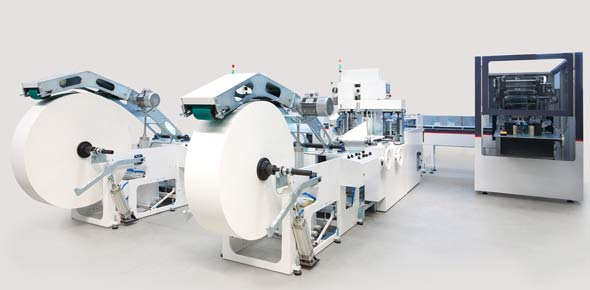Simple Machines Ch 14

15 Questions | Total Attempts: 40Settings• 1.
The purpose of a simple machine to make work easier by allowing for a push or pull to occur over an increased distance.
• A.

True

• B.

False

• 2.
What is a simple machine that uses grooved wheels and a rope to raise, lower, or move a load?
• A.

Lever

• B.

Inclined plane

• C.

Wheel and axel

• D.

Pulley

• 3.
What simple machine uses a wheel with a rod or rods through its center to help it move a load?
• A.

Inclined plane

• B.

Wheel and axel

• C.

Screw

• D.

All of the abouve

• 4.
What simple machine uses a slanted surface that connects a lower level to a higher level?
• A.

Inclined plane

• B.

Screw

• C.

Wedge

• D.

Lever

• 5.
What type of simple machine is a seesaw on a playground?
• A.

Inclined plane

• B.

Pulley

• C.

Wedge

• D.

Lever

• 6.
What type of simple machine would the lid of a jar be?
• A.

Screw

• B.

Wedge

• C.

Lever

• D.

Pulley

• 7.
An axe would be what type of simple machine?
• A.

Pulley

• B.

Wedge

• C.

Lever

• D.

Screw

• 8.
Which one do you like?
• A.

Option 1

• B.

Option 2

• C.

Option 3

• D.

Option 4

• 9.
A compound machine is two or more simple machines that are working together to make work easier.
• A.

True

• B.

False

• 10.
If the mechanical advantage of a simple machine is 4, then the
• A.

Output force is 4 times the effort

• B.

Effort is 4 times the output force

• C.

Efficiency is 4%

• D.

The work output is 4 times the input

• 11.
The fixed point on a lever is a
• A.

Triangle

• B.

Fulcrum

• C.

Function

• D.

Gear

• 12.
What is an example of a wheel and axle?
• A.

Screwdriver

• B.

Wrench

• C.

Door knob

• D.

Hammer

• 13.
How much work does a 25 newton force do to lift a potted plant from the floor to a shelf 1.5 meters high?
• 14.
A truck pulls a trailer at a constant velocity for 100 m while exerting a force of 480 newtons for 60 seconds.  Calculate the power.
• 15.
In order for work to be done you need _________________ .
Related TopicsBack to top